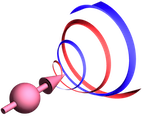#Löffler Lab

Quantum Matter and Optics
Huygens Laboratory
Leiden University, NL

## Photon (etc) units & bandwidth

Speed of wave, defaults to light (m/s):

Absolute quantities:

 wavelength (nm): energy (eV): frequency (THz): wavenumber (cm-1): temperature (K):

Relative to above:

 wavelength (nm): energy (eV): frequency (THz): wavenumber (cm-1): temperature (K): pulse duration (ps): pulse length (m): cavity round trip length (m):

Pulse is assumed to be transform limited Gaussian (TBP=0.44).
Temperature is from E=k_B T.
Round trip cavity length is in vacuum with FSR=relative frequency.

## High-finesse Fabry-Perot cavity:

 mirror reflectivity R1: cavity finesse (high R1, R2=R1): mirror reflectivity R2: cavity finesse (high R1,R2): single mirror scattering+absorption loss: on-resonance transmission: on-resonance reflection: